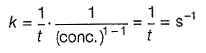Courses

# Retro (Past 13 Year) JEE Main (Chemical Kinetics)

## 28 Questions MCQ Test Chemistry for JEE Advanced | Retro (Past 13 Year) JEE Main (Chemical Kinetics)

Description
This mock test of Retro (Past 13 Year) JEE Main (Chemical Kinetics) for JEE helps you for every JEE entrance exam. This contains 28 Multiple Choice Questions for JEE Retro (Past 13 Year) JEE Main (Chemical Kinetics) (mcq) to study with solutions a complete question bank. The solved questions answers in this Retro (Past 13 Year) JEE Main (Chemical Kinetics) quiz give you a good mix of easy questions and tough questions. JEE students definitely take this Retro (Past 13 Year) JEE Main (Chemical Kinetics) exercise for a better result in the exam. You can find other Retro (Past 13 Year) JEE Main (Chemical Kinetics) extra questions, long questions & short questions for JEE on EduRev as well by searching above.
QUESTION: 1

### Higher order (>3) reactions are rare due to

Solution:

The probability that more than three molecules can collide and react simultaneously is very small. Thus, order of the reaction greater than three is very rare.

QUESTION: 2

### For the non-stoichiometric reaction,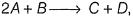the following kinetic data were obtained in three separate experiments, all at 298 K.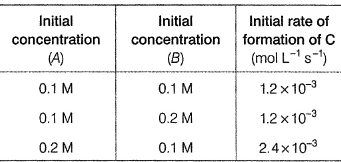Q.  The rate law for the formation of C is (JEE Main 2014)

Solution:

Rate law equation is given by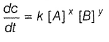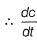for C at various concentrations are
1.2 x 10-3 = k(0.1)x(0.1)y  .....(i)
1.2 x 10-3 = k(0.1)x(0.2)y ......(ii)
2.4 x 10-3 = k(0.2)x(0.1)y  ......(iii)
Solving for x [divide Eq. (iii) by Eq. (i)]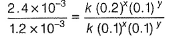2 = (2 )x
⇒ x = 1
Solving for y [divide Eq. (iii) by Eq. (i)]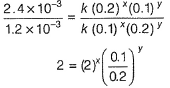Put x = 1 in above equation, we get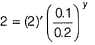1 = (0.5)y
(1)° = (0.5)y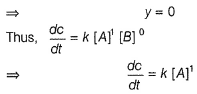QUESTION: 3

### The rate of a reaction double when its temperature changes from 300 K to 310 K. Activation energy of such a reaction will be (given,R = 8.314 JK-1 mol-1 and log 2 = 0.301) (JEE Main 2013)

Solution:

From Arrhenius equation,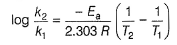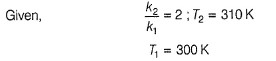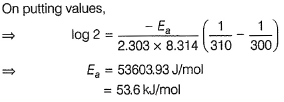QUESTION: 4

For a first order reaction, (A)→ products the concentration of A changes from 0.1 M to 0.025 Min 40 min. The rate of reaction when the concentration of A is 0.01 M is

(AiEEE 2012)

Solution:

Rate constant for the first order reaction is given by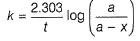a = 0.1 M
(a - x) = 0.025 M
t = 40 min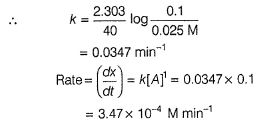QUESTION: 5

The rate of a chemical reaction doubles for every 10°C rise of temperature. If the temperature is raised by 50°C, the rate of the reaction increases by about

(AIEEE 2011)

Solution:

For every 10°C rise of temperature, rate is doubled. Thus, temperature coefficient of the reaction = 2
When temperature is increased by 50°, rate becomes
= 2 (50/10) = 25 times = 32 times

QUESTION: 6

A reactant (A) forms two products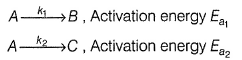If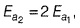then k1 and k2 are related as

(AIEEE 2011)

Solution: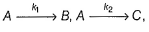By Arrhenius equation,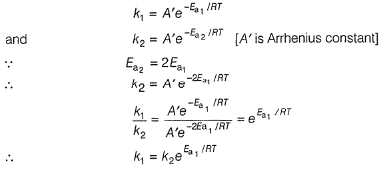QUESTION: 7

The time for half-life period of a certain reaction, A → products is 1 h, when the initial concentration of the reactant ‘A’ is 2.0 mol L-1. How much time does it take for its concentration to come from 0.50 to 0.25 mol L-1, if it is a zero order reaction?

(AIEEE 2010)

Solution:

Half- life for a zero order reaction is given by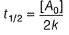where [A0] = initial concentration of reactant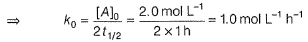Rate constant for a zero order reaction is given by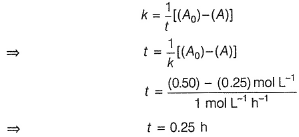QUESTION: 8

Consider the reaction,
Cl2(aq) + H2S(ag) → S(s) + 2H+ (aq) + 2CI- (aq)
The rate equation for this reaction is,
rate =k[Cl2] [H2S]

Q.

Which of these mechanisms is/are consistent with this rate equation?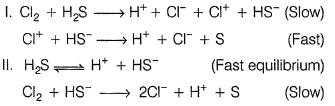(AIEEE 2010)

Solution:

Slowest step is the rate determining step . Thus, in case (I), rate law is given as rate = k[CI2] [H2S]
While for the reaction given in case (II), rate law is given as rate
= k[H2S ][CI2] [H+]-1.
Hence, only mechanism (I) is consistent with the given rate law.

QUESTION: 9

The half-life period of a first order chemical reaction is 6.93 min. The time required for the completion of 99% of the chemical reaction will be (take, log 2= 0.301)

(AIEEE 2009)

Solution:

Half-life = 6.93 min,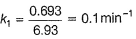Rate constant for the first order reaction is given by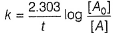where, [A0] = initial concentration
[A] = final concentration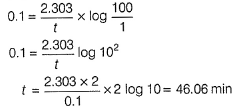QUESTION: 10

For a reaction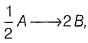rate of disappearance of A is related to the rate of appearance of B by the expression

(AIEEE 2008)

Solution:Remember for aA → bB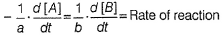For the given reaction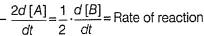Rate of disappearance of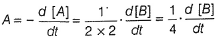QUESTION: 11

Consider the reaction, 2A + B → product. When concentration of B alone was doubled, the half-life did not change. When the concentration. of A alone was doubled, the rate increased by two times. The unit of rate constant for this reaction is

(AIEEE 2007)

Solution:

(a) 2 A + 6 → Products
[B] is doubled, half-life didn’t change.
Half-life is independent of change in concentration of reactant i.e. first order
First order w.r.t. B
When [A] is doubled, rate increased by two times.
⇒ First order w.r.t. A
Hence, net order of reaction = 1 + 1 = 2
Unit for the rate constant = conc(1- n) -1
= (mol L-1)-1. s-1
= Lmol-1 s-1

QUESTION: 12

The energies of activation for forward and reverse reactions for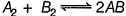are 180 kJ mol-1 and 200 kJ mol-1, respectively. The presence of a catalyst lowers the activation energy of both (forward and reverse) reactions by 100 kJ mol-1. The enthalpy change of the reaction (A2 + B2 → 2 AB) in the presence of catalyst will be (in kJ mol-1)

(AIEEE 2007)

Solution:Ea (forward) = 180 kJ mol-1
Ea (backward) = 200 kJ mol-1
In the presence of catalyst,
Ea (forward) = 180 - 100 = 80 kJ mol-1
Ea (backward) = 200 - 100 = 100 kJ mol-1
ΔH = Ea (forward) - Ea (backward)
= 80 - 100
= - 20 kJ mol-1
For the value of enthalpy change of the reaction, negative sign can be neglected.

QUESTION: 13

A radioactive element gets spilled over the floor of a room. Its half-life period is 30 days. If the initial activity is ten times the permissible value, after how many days will it be safe to enter the room?

(AIEEE 2007)

Solution: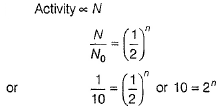Taking log on both sides
log 10 = n log 2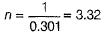Time = n x half-life = 3.32 x 30
= 99.6 days
= 100 days

QUESTION: 14

The following mechanism has been proposed for the reaction of NO with Br2 to form NOBr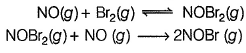Q.

If the second step is the rate determining step, the order of the reaction with respect to NO (g) is

(AIEEE 2006)

Solution:

Rate = k[NOBr2] [NO]     ......(i)
But NOBr2 is in equilibrium.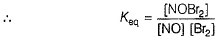[NOBr2] = Keq [NO] [Br2]
Putting the [NOBr2] in Eq. (i), we get
Rate = k-Keq [NO] [Br2] [NO]
Hence, Rate = k . Keq [NO]2 [Br2]
Rate = k' [NO]2 [Br2]
where k' = k- Keq
Thus, order w.r.t. NO is 2.

QUESTION: 15

Rate of a reaction can be expressed by Arrhenius equation as k = Ae-E/RT In this equation, E represents

(AIEEE 2006)

Solution:

According to Arrhenius equation
k = Ae-E/RT
where, E is the activation energy below which the colliding molecules will not react.

QUESTION: 16

A reaction was found to be second order with respect to the concentration of carbon monoxide. If the concentration of carbon monoxide is doubled, with everything else kept the same, the rate of reaction will

(AIEEE 2006)

Solution:

If order = n then on making concentration m-times, rate becomes mn times
n = 2 , m = 2
Thus, new rate = 22 = 4 times

QUESTION: 17

A reaction involving two different reactants can never be

(AIEEE 2005)

Solution:

There are two different reactants (say A and B).
A + B → product
Thus, it is a bimolecular reaction.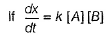It is second order reaction.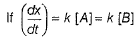It is first order reaction.
Molecularity is independent of rate but is the sum of the reacting substances. Thus, it cannot be unimolecular reaction.

QUESTION: 18

t1/4 can be taken as the time taken for the concentration of a reactant to drop to 3/4 of its initial value. If the rate constant for a first order reaction is k1 the t1/4 can be written as

(AIEEE 2005)

Solution:

A → Product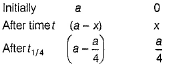For first order kinetics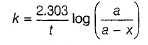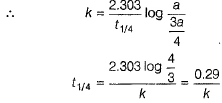QUESTION: 19

Consider an e ndotherm ic reaction X → V with the activation energies Eb and Ef for the backward and forward reactions respectively. In general

(AIEEE 2005)

Solution:

X → Y is an endothemric reaction, ΔH = + ve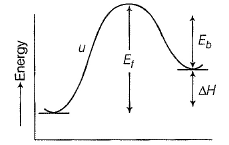Eb = energy of activation of backward reaction
Ef = energy of activation of forward reaction
ΔH = heat of reaction
Thus, E1 = Eb + ΔH, E1 > Eb

QUESTION: 20

The rate equation for the reaction 2A + B → C is found to be rate = k [A] [B]

The correct statement in relation to this reaction is that the

(AIEEE 2004)

Solution:

2A + B → C
Rate = k [A] [B]
It represents second order reaction.
Thus, unit of k is L mol-1 s-1.
Hence, (a) is false,
t1/2 is dependent of concentration but not constant.
Hence, (b) is false.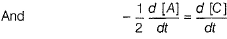Thus, (c) is also false,
unit of k = conc1-x time-1
By rate law n = 2
Thus, unit is cone-1 time-1
(L mol-1 s-1 if concentration in mol L-1 and time in second)

QUESTION: 21

In a first order reaction, the concentration of the reactant, decreases from 0.8 M to 0.4 M in 15 min. The time taken for the concentration to change from 0.1 M to 0.025 M is

(AIEEE 2004)

Solution:

Order = 1
Concentration changes from 0.8 M to 0.4 M in (50%).
15 min, thus half-life = 15 min = T50
A change from 0.1 M to 0.025 is 75% and for first order reaction
T75 = 2 x T50 = 2 x 15 = 30 min
Alternate Method
T50 = 15 min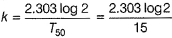a = 0.1 M
(a - x) = 0.025 M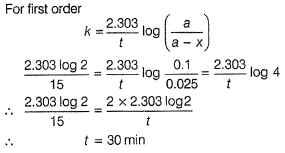QUESTION: 22

In respect of the equation k = Ae - Ea/RT in chemical kinetics, which one of the following statements is correct?

(AIEEE 2003)

Solution:

By Arrhenius equation,
k = Ae-Ea/RT, k = Rate constant
A = Pre-exponential, frequency factor
Ea = Activation energy, R = Gas constant
T = Temperature

QUESTION: 23

The rate law for a reaction between the substances A and B is given by rate = k [A]n [B]m. On doubling the concentration of A and halving the concentration of B, the ratio of the new rate to the earlier rate of the reaction will be as

(AIEEE 2003)

Solution:

Rate, r = k [A]n [B]m
On doubling [A] and halving [B], the rate becomes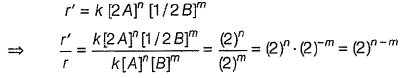QUESTION: 24

For the reaction system,

2NO (g) + O2(g) → 2NO2(g)

volume is suddenly reduced to half its value by increasing the pressure on it. If the reaction is of first order with respect to O2 and second order with respect to NO, the rate of reaction will

(AIEEE 2003)

Solution: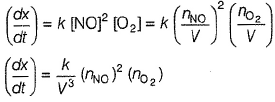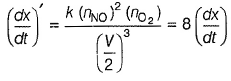QUESTION: 25

For the reaction, H2 + l2 → 2HI, the differential rate law is

(AIEEE 2002)

Solution: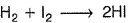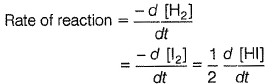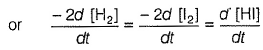QUESTION: 26

Rate constant, k of the first order reaction when initial concentration (C0) and concentration (Ct)at time t, is given by equation kt = log C0 - log Ct Graph is a straight line, if we plot

(AIEEE 2002)

Solution: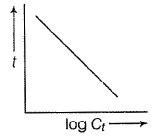kt = - loge Ct + loge C0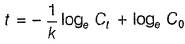y = mx + c, straight line, negative slope.

QUESTION: 27

For a reaction A + 2B→ C, rate is given by +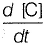= k [A] [B], hence the order of the reaction is

(AIEEE 2002)

Solution:

Rate of the reaction depend upon the concentration of A and 8 both, hence it is a second order reaction.

QUESTION: 28

Consider the following two reactions,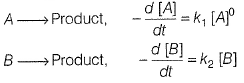k1 and k2 are expressed in terms of molarity (mol L-1)and time (s-1)as

(AIEEE 2002)

Solution:

Unit of rate constant,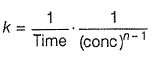For k1, n = 0,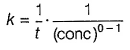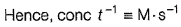For k2, n = 1